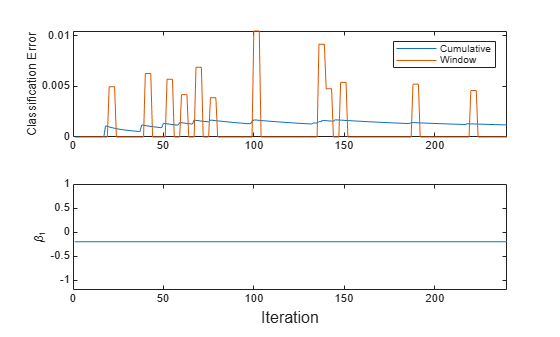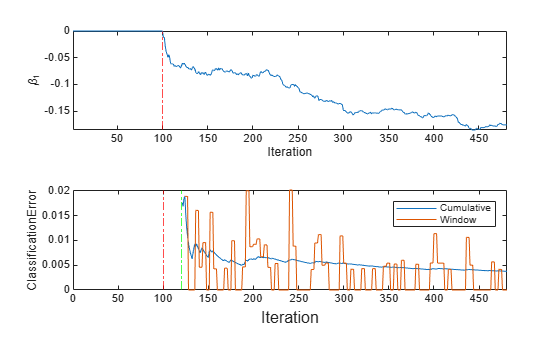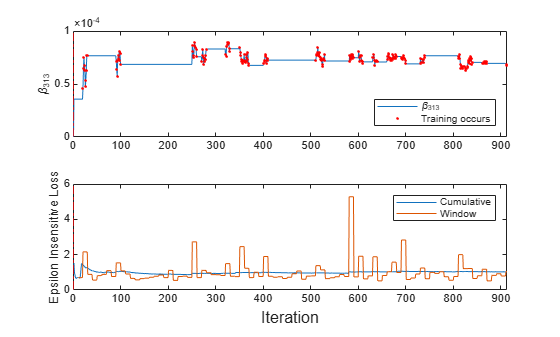# updateMetrics

Update performance metrics in linear model for incremental learning given new data

## Syntax

Mdl = updateMetrics(Mdl,X,Y)
Mdl = updateMetrics(Mdl,X,Y,Name,Value)

## Description

Given streaming data, updateMetrics measures the performance of a configured incremental learning model for linear regression (incrementalRegressionLinear object) or linear binary classification (incrementalClassificationLinear object). updateMetrics stores the performance metrics in the output model.

updateMetrics allows for flexible incremental learning. After you call the function to update model performance metrics on an incoming chunk of data, you can perform other actions before you train the model to the data. For example, you can decide whether you need to train the model based on its performance on a chunk of data. Alternatively, you can both update model performance metrics and train the model on the data as it arrives, in one call, by using the updateMetricsAndFit function.

To measure the model performance on a specified batch of data, call loss instead.

example

Mdl = updateMetrics(Mdl,X,Y) returns an incremental learning model Mdl, which is the input incremental learning model Mdl modified to contain the model performance metrics on the incoming predictor and response data, X and Y respectively.When the input model is warm (Mdl.IsWarm is true), updateMetrics overwrites previously computed metrics, stored in the Metrics property, with the new values. Otherwise, updateMetrics stores NaN values in Metrics instead.The input and output models have the same data type.

example

Mdl = updateMetrics(Mdl,X,Y,Name,Value) uses additional options specified by one or more name-value pair arguments. For example, you can specify that the columns of the predictor data matrix correspond to observations, and set observation weights.

## Examples

collapse all

Train a linear model for binary classification by using fitclinear, convert it to an incremental learner, and then track its performance to streaming data.

Load the human activity data set. Randomly shuffle the data.

load humanactivity rng(1); % For reproducibility n = numel(actid); idx = randsample(n,n); X = feat(idx,:); Y = actid(idx);

For details on the data set, enter Description at the command line.

Responses can be one of five classes: Sitting, Standing, Walking, Running, or Dancing. Dichotomize the response by identifying whether the subject is moving (actid > 2).

Y = Y > 2;

Train Linear Model for Binary Classification

Fit a linear model for binary classification to a random sample of half the data.

idxtt = randsample([true false],n,true); TTMdl = fitclinear(X(idxtt,:),Y(idxtt))
TTMdl = ClassificationLinear ResponseName: 'Y' ClassNames: [0 1] ScoreTransform: 'none' Beta: [60x1 double] Bias: -0.3965 Lambda: 8.2967e-05 Learner: 'svm' Properties, Methods 

TTMdl is a ClassificationLinear model object representing a traditionally trained linear model for binary classification.

Convert Trained Model

Convert the traditionally trained classification model to a binary classification linear model for incremental learning.

IncrementalMdl = incrementalLearner(TTMdl)
IncrementalMdl = incrementalClassificationLinear IsWarm: 1 Metrics: [1x2 table] ClassNames: [0 1] ScoreTransform: 'none' Beta: [60x1 double] Bias: -0.3965 Learner: 'svm' Properties, Methods 
IncrementalMdl.IsWarm
ans = logical 1 

The incremental model is warm. Therefore, updateMetrics can track model performance metrics given data.

Track Performance Metrics

Track the model performance on the rest of the data by using the updateMetrics function. Simulate a data stream by processing 50 observations at a time. At each iteration:

1. Call updateMetrics to update the cumulative and window classification error of the model given the incoming chunk of observations. Overwrite the previous incremental model to update the losses in the Metrics property. Note that the function does not fit the model to the chunk of data—the chunk is "new" data for the model.

2. Store the classification error and first coefficient ${\beta }_{1}$.

% Preallocation idxil = ~idxtt; nil = sum(idxil); numObsPerChunk = 50; nchunk = floor(nil/numObsPerChunk); ce = array2table(zeros(nchunk,2),'VariableNames',["Cumulative" "Window"]); beta1 = [IncrementalMdl.Beta(1); zeros(nchunk,1)]; Xil = X(idxil,:); Yil = Y(idxil); % Incremental fitting for j = 1:nchunk ibegin = min(nil,numObsPerChunk*(j-1) + 1); iend = min(nil,numObsPerChunk*j); idx = ibegin:iend; IncrementalMdl = updateMetrics(IncrementalMdl,Xil(idx,:),Yil(idx)); ce{j,:} = IncrementalMdl.Metrics{"ClassificationError",:}; beta1(j + 1) = IncrementalMdl.Beta(1); end

IncrementalMdl is an incrementalClassificationLinear model object that has tracked the model performance to observations in the data stream.

Plot a trace plot of the performance metrics and estimated coefficient ${\beta }_{1}$.

figure; subplot(2,1,1) h = plot(ce.Variables); xlim([0 nchunk]); ylabel('Classification Error') legend(h,ce.Properties.VariableNames) subplot(2,1,2) plot(beta1) ylabel('\beta_1') xlim([0 nchunk]); xlabel('Iteration')The cumulative loss is stable, whereas the window loss jumps.

${\beta }_{1}$ does not change because updateMetrics does not fit the model to the data.

Create a incremental linear SVM model for binary classification. Specify an estimation period of 5,000 observations and the SGD solver.

Mdl = incrementalClassificationLinear('EstimationPeriod',5000,'Solver','sgd')
Mdl = incrementalClassificationLinear IsWarm: 0 Metrics: [1x2 table] ClassNames: [1x0 double] ScoreTransform: 'none' Beta: [0x1 double] Bias: 0 Learner: 'svm' Properties, Methods 

Mdl is an incrementalClassificationLinear model. All its properties are read-only.

Determine whether the model is warm and the size of the metrics warm-up period by querying model properties.

isWarm = Mdl.IsWarm
isWarm = logical 0 
mwp = Mdl.MetricsWarmupPeriod
mwp = 1000 

Mdl.IsWarm is 0; therefore, Mdl is not warm.

Determine the number of observations incremental fitting functions, such as fit, must process before measuring the performance of the model.

numObsBeforeMetrics = Mdl.MetricsWarmupPeriod + Mdl.EstimationPeriod
numObsBeforeMetrics = 6000 

Load the human activity data set. Randomly shuffle the data.

load humanactivity n = numel(actid); rng(1) % For reproducibility idx = randsample(n,n); X = feat(idx,:); Y = actid(idx);

For details on the data set, enter Description at the command line.

Responses can be one of five classes: Sitting, Standing, Walking, Running, or Dancing. Dichotomize the response by identifying whether the subject is moving (actid > 2).

Y = Y > 2;

Perform incremental learning. At each iteration, perform the following actions:

• Simulate a data stream by processing a chunk of 50 observations.

• Measure model performance metrics on the incoming chunk using updateMetrics. Overwrite the input model.

• Fit the model to the incoming chunk. Overwrite the input model.

• Store ${\beta }_{1}$ and the misclassification error rate to see how they evolve during incremental learning.

% Preallocation numObsPerChunk = 50; nchunk = floor(n/numObsPerChunk); ce = array2table(zeros(nchunk,2),'VariableNames',["Cumulative" "Window"]); beta1 = zeros(nchunk,1); % Incremental fitting for j = 1:nchunk ibegin = min(n,numObsPerChunk*(j-1) + 1); iend = min(n,numObsPerChunk*j); idx = ibegin:iend; Mdl = updateMetrics(Mdl,X(idx,:),Y(idx)); ce{j,:} = Mdl.Metrics{"ClassificationError",:}; Mdl = fit(Mdl,X(idx,:),Y(idx)); beta1(j) = Mdl.Beta(1); end

IncrementalMdl is an incrementalClassificationLinear model object trained on all the data in the stream.

To see how the parameters evolved during incremental learning, plot them on separate subplots.

figure; subplot(2,1,1) plot(beta1) ylabel('\beta_1') xline(Mdl.EstimationPeriod/numObsPerChunk,'r-.'); xlabel('Iteration') axis tight subplot(2,1,2) plot(ce.Variables); ylabel('ClassificationError') xline(Mdl.EstimationPeriod/numObsPerChunk,'r-.'); xline(numObsBeforeMetrics/numObsPerChunk,'g-.'); xlabel('Iteration') xlim([0 nchunk]); legend(ce.Properties.VariableNames)mdlIsWarm = numObsBeforeMetrics/numObsPerChunk
mdlIsWarm = 120 

The plot suggests that fit does not fit the model to the data or update the parameters until after the estimation period. Also, updateMetrics does not track the classification error until after the estimation and metrics warm-up periods (120 chunks).

Incrementally train a linear regression model only when its performance degrades.

Load and shuffle the 2015 NYC housing data set. For more details on the data, see NYC Open Data.

load NYCHousing2015 rng(1) % For reproducibility n = size(NYCHousing2015,1); shuffidx = randsample(n,n); NYCHousing2015 = NYCHousing2015(shuffidx,:);

Extract the response variable SALEPRICE from the table. For numerical stability, scale SALEPRICE by 1e6.

Y = NYCHousing2015.SALEPRICE/1e6; NYCHousing2015.SALEPRICE = [];

Create dummy variable matrices from the categorical predictors.

catvars = ["BOROUGH" "BUILDINGCLASSCATEGORY" "NEIGHBORHOOD"]; dumvarstbl = varfun(@(x)dummyvar(categorical(x)),NYCHousing2015,... 'InputVariables',catvars); dumvarmat = table2array(dumvarstbl); NYCHousing2015(:,catvars) = [];

Treat all other numeric variables in the table as linear predictors of sales price. Concatenate the matrix of dummy variables to the rest of the predictor data, and transpose the data to speed up computations.

idxnum = varfun(@isnumeric,NYCHousing2015,'OutputFormat','uniform'); X = [dumvarmat NYCHousing2015{:,idxnum}]';

Configure a linear regression model for incremental learning so that it does not have an estimation or metrics warm-up period. Specify a metrics window size of 1000 observations. Fit the configured model to the first 100 observations, and specify that the observations are oriented along the columns of the data.

Mdl = incrementalRegressionLinear('EstimationPeriod',0,'MetricsWarmupPeriod',0,... 'MetricsWindowSize',1000); numObsPerChunk = 100; Mdl = fit(Mdl,X(:,1:numObsPerChunk),Y(1:numObsPerChunk),'ObservationsIn','columns');

Mdl is an incrementalRegressionLinear model object.

Perform incremental learning, with conditional fitting, by following this procedure for each iteration:

• Simulate a data stream by processing a chunk of 100 observations.

• Update the model performance by computing the epsilon insensitive loss, within a 200 observation window. Specify that the observations are oriented along the columns of the data.

• Fit the model to the chunk of data only when the loss more than doubles from the minimum loss experienced. Specify that the observations are oriented along the columns of the data.

• When tracking performance and fitting, overwrite the previous incremental model.

• Store the epsilon insensitive loss and ${\beta }_{313}$ to see the how the loss and coefficient evolve during training.

• Track when fit trains the model.

% Preallocation n = numel(Y) - numObsPerChunk; nchunk = floor(n/numObsPerChunk); beta313 = zeros(nchunk,1); ei = array2table(nan(nchunk,2),'VariableNames',["Cumulative" "Window"]); trained = false(nchunk,1); % Incremental fitting for j = 2:nchunk ibegin = min(n,numObsPerChunk*(j-1) + 1); iend = min(n,numObsPerChunk*j); idx = ibegin:iend; Mdl = updateMetrics(Mdl,X(:,idx),Y(idx),'ObservationsIn','columns'); ei{j,:} = Mdl.Metrics{"EpsilonInsensitiveLoss",:}; minei = min(ei{:,2}); pdiffloss = (ei{j,2} - minei)/minei*100; if pdiffloss > 100 Mdl = fit(Mdl,X(:,idx),Y(idx),'ObservationsIn','columns'); trained(j) = true; end beta313(j) = Mdl.Beta(end); end

Mdl is an incrementalRegressionLinear model object trained on all the data in the stream.

To see how the model performance and ${\beta }_{313}$ evolved during training, plot them on separate subplots.

subplot(2,1,1) plot(beta313) hold on plot(find(trained),beta313(trained),'r.') ylabel('\beta_{313}') xline(Mdl.EstimationPeriod/numObsPerChunk,'r-.'); legend('\beta_{313}','Training occurs','Location','southeast') hold off subplot(2,1,2) plot(ei.Variables) ylabel('Epsilon Insensitive Loss') xline(Mdl.EstimationPeriod/numObsPerChunk,'r-.'); xlabel('Iteration') legend(ei.Properties.VariableNames)The trace plot of ${\beta }_{313}$ shows periods of constant values, during which the loss did not double from the minimum experienced.

## Input Arguments

collapse all

Incremental learning model whose performance is measured, specified as an incrementalClassificationLinear or incrementalRegressionLinear model object. You can create Mdl directly or by converting a supported, traditionally trained machine learning model using the incrementalLearner function. For more details, see the corresponding reference page.

If Mdl.IsWarm is false, updateMetrics does not track the performance of the model. You must fit Mdl to Mdl.EstimationPeriod + Mdl.MetricsWarmupPeriod observations by passing Mdl and the data to fit before updateMetrics can track performance metrics. For more details, see Algorithms.

Chunk of predictor data with which to measure the model performance, specified as a floating-point matrix of n observations and Mdl.NumPredictors predictor variables. The value of the 'ObservationsIn' name-value pair argument determines the orientation of the variables and observations.

The length of the observation labels Y and the number of observations in X must be equal; Y(j) is the label of observation j (row or column) in X.

Note

• If Mdl.NumPredictors = 0, updateMetrics infers the number of predictors from X, and sets the congruent property of the output model. Otherwise, if the number of predictor variables in the streaming data changes from Mdl.NumPredictors, updateMetrics issues an error.

• updateMetrics supports only floating-point input predictor data. If the input model Mdl represents a converted, traditionally trained model fit to categorical data, use dummyvar to convert each categorical variable to a numeric matrix of dummy variables, and concatenate all dummy variable matrices and any other numeric predictors. For more details, see Dummy Variables.

Data Types: single | double

Chunk of labels with which to measure the model performance, specified as a categorical, character, or string array, logical or floating-point vector, or cell array of character vectors for classification problems; or a floating-point vector for regression problems.

The length of the observation labels Y and the number of observations in X must be equal; Y(j) is the label of observation j (row or column) in X.

For classification problems:

• updateMetrics supports binary classification only.

• When the ClassNames property of the input model Mdl is nonempty, the following conditions apply:

• If Y contains a label that is not a member of Mdl.ClassNames, updateMetrics issues an error.

• The data type of Y and Mdl.ClassNames must be the same.

Data Types: char | string | cell | categorical | logical | single | double

Note

If an observation (predictor or label) or weight Weight contains at least one missing (NaN) value, updateMetrics ignores the observation. Consequently, updateMetrics uses fewer than n observations to compute the model performance.

### Name-Value Arguments

Specify optional comma-separated pairs of Name,Value arguments. Name is the argument name and Value is the corresponding value. Name must appear inside quotes. You can specify several name and value pair arguments in any order as Name1,Value1,...,NameN,ValueN.

Example: 'ObservationsIn','columns','Weights',W specifies that the columns of the predictor matrix correspond to observations, and the vector W contains observation weights to apply during incremental learning.

Predictor data observation dimension, specified as the comma-separated pair consisting of 'ObservationsIn' and 'columns' or 'rows'.

Data Types: char | string

Chunk of observation weights, specified as the comma-separated pair consisting of 'Weights' and a floating-point vector of positive values. updateMetrics weighs the observations in X with the corresponding values in Weights. The size of Weights must equal n, which is the number of observations in X.

By default, Weights is ones(n,1).

For more details, including normalization schemes, see Observation Weights.

Data Types: double | single

## Output Arguments

collapse all

Updated incremental learning model, returned as an incremental learning model object of the same data type as the input model Mdl, either incrementalClassificationLinear or incrementalRegressionLinear.

If the model is not warm, updateMetrics does not compute performance metrics. As a result, the Metrics property of Mdl remains completely composed of NaN values. If the model is warm, updateMetrics computes the cumulative and window performance metrics on the new data X and Y, and overwrites the corresponding elements of Mdl.Metrics. All other properties of the input model Mdl carry over to the output model Mdl. For more details, see Algorithms.

## Tips

• Unlike traditional training, incremental learning might not have a separate test (holdout) set. Therefore, to treat each incoming chunk of data as a test set, pass the incremental model and each incoming chunk to updateMetrics before training the model on the same data using fit.

## Algorithms

collapse all

### Performance Metrics

• updateMetrics tracks only model performance metrics, specified by the row labels of the table in Mdl.Metrics, from new data when the incremental model is warm (IsWarm property is true). An incremental model is warm after the fit function fits the incremental model to Mdl.MetricsWarmupPeriod observations, which is the metrics warm-up period.

If Mdl.EstimationPeriod > 0, the functions estimate hyperparameters before fitting the model to data. Therefore, the functions must process an additional EstimationPeriod observations before the model starts the metrics warm-up period.

• The Metrics property of the incremental model stores two forms of each performance metric as variables (columns) of a table, Cumulative and Window, with individual metrics in rows. When the incremental model is warm, updateMetrics updates the metrics at the following frequencies:

• Cumulative — The function computes cumulative metrics since the start of model performance tracking. The function updates metrics every time you call it and bases the calculation on the entire supplied data set.

• Window — The function computes metrics based on all observations within a window determined by the Mdl.MetricsWindowSize property. Mdl.MetricsWindowSize also determines the frequency at which the software updates Window metrics. For example, if Mdl.MetricsWindowSize is 20, the function computes metrics based on the last 20 observations in the supplied data (X((end – 20 + 1):end,:) and Y((end – 20 + 1):end)).

Incremental functions that track performance metrics within a window use the following process:

1. For each specified metric, store a buffer of length Mdl.MetricsWindowSize and a buffer of observation weights.

2. Populate elements of the metrics buffer with the model performance based on batches of incoming observations, and store corresponding observations weights in the weights buffer.

3. When the buffer is filled, overwrite Mdl.Metrics.Window with the weighted average performance in the metrics window. If the buffer is overfilled when the function processes a batch of observations, the latest incoming Mdl.MetricsWindowSize observations enter the buffer, and the earliest observations are removed from the buffer. For example, suppose Mdl.MetricsWindowSize is 20, the metrics buffer has 10 values from a previously processed batch, and 15 values are incoming. To compose the length 20 window, the function uses the measurements from the 15 incoming observations and the latest 5 measurements from the previous batch.

### Observation Weights

For classification problems, if the prior class probability distribution is known (in other words, the prior distribution is not empirical), updateMetrics normalizes observation weights to sum to the prior class probabilities in the respective classes. This action implies that observation weights are the respective prior class probabilities by default.

For regression problems or if the prior class probability distribution is empirical, the software normalizes the specified observation weights to sum to 1 each time you call updateMetrics.

## Extended Capabilities

Introduced in R2020b